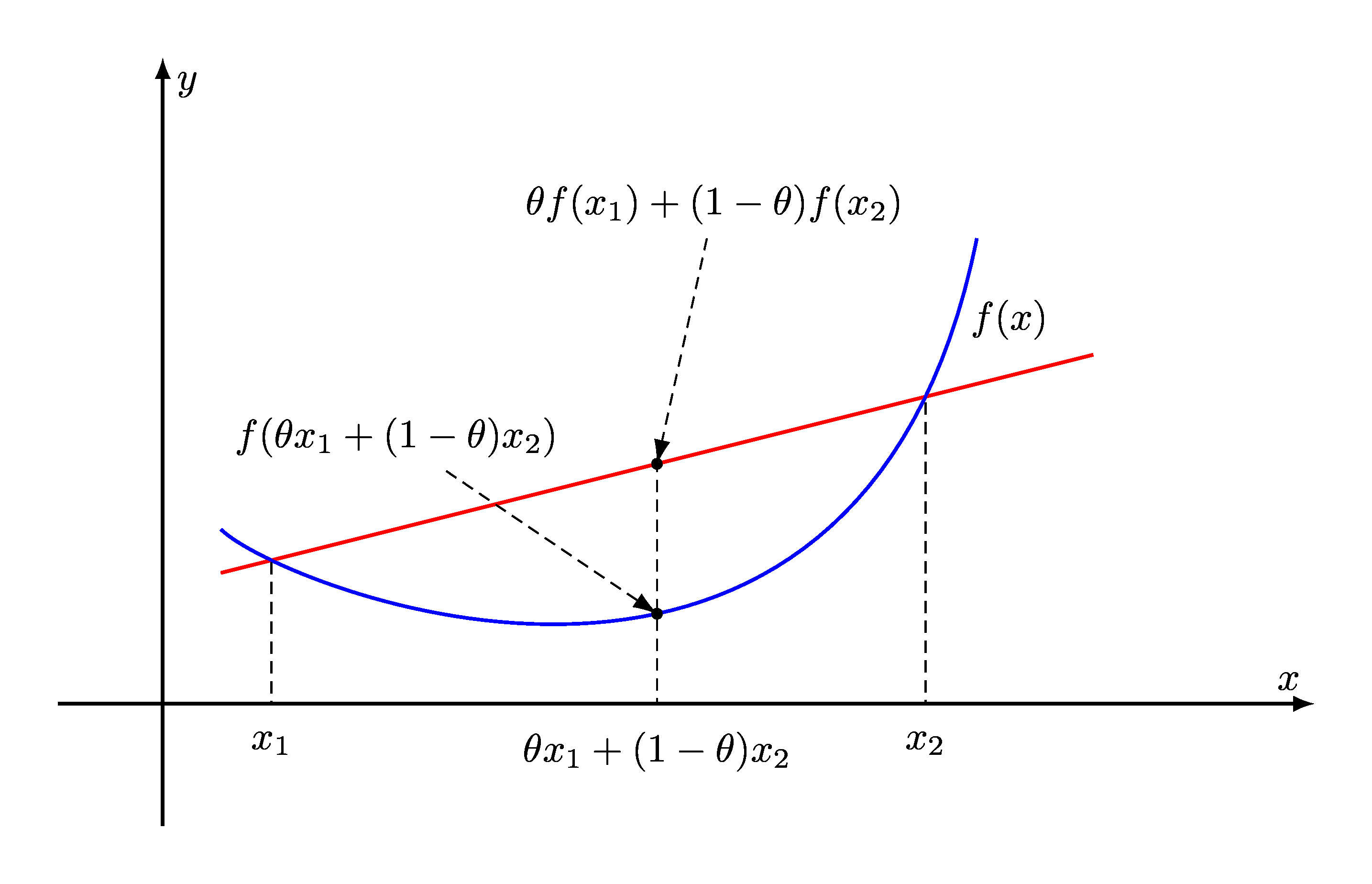# 10. Convex Optimization#

This chapter provides general introduction to convex optimization problems. Convex optimization focuses on a special class of mathematical optimization problems where:

• The real valued function being optimized (maximized or minimized) is convex.

• The feasible set of values for the function is a closed convex set.Fig. 10.1 For a convex function, the line segment between any two points on the graph of the function does not lie below the graph between the two points. Its epigraph is a convex set. A local minimum of a convex function is also a global minimum.#

Convex optimization problems are usually further classified into

• Least squares

• Linear programming

• Quadratic minimization with linear constraints

• Quadratic minimization with convex quadratic constraints

• Conic optimization

• Geometric programming

• Second order cone programming

• Semidefinite programming

There are specialized algorithms available for each of these classes.

Some of the applications of convex optimization include:

• Portfolio optimization

• Worst case risk analysis

• Compressive sensing

• Statistical regression

• Model fitting

• Combinatorial Optimization